# Functions Set 2

Go back to  'SOLVED EXAMPLES'

Example – 3

Find the roots of the function $$f(x) = a{x^2} + bx + c$$  and plot its graph. Also solve the inequalities  $$f(x) \ge 0$$ and $$f(x) \le 0$$

Solution: The expression for   $$f(x)$$  is quadratic, of which we have seen some examples. Lets discuss a particular case for  $$f(x)$$ and then go on to the general case above.

Let   $$f(x) = 3{x^2} + 10x + 3$$  which can be written as $$f(x) = (3x + 1)(x + 3).$$  This is 0 when either    $$3x + 1 = 0$$  or   $$x + 3 = 0$$

$i.e.\qquad \qquad {\rm{ }}x = - \frac{1}{3},{\mkern 1mu} {\mkern 1mu} - 3{\rm{ }}\qquad \qquad\qquad ...(i)$

Now                $$f(x) = 3{x^2} + 10x + 3$$    can be rearranged as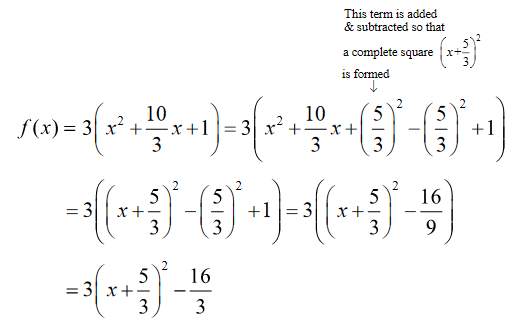We can obtain the graph for f(x) in the following steps: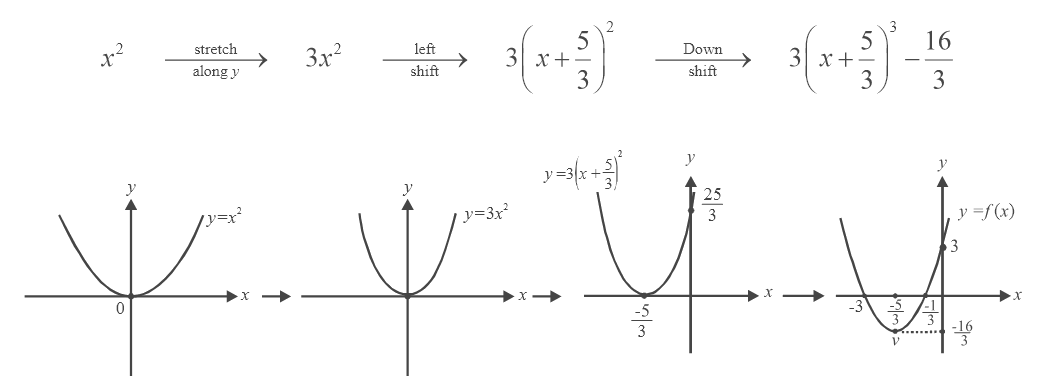We see that the coordinates of the vertex (the point v) are \begin{align}\left( { - \frac{5}{3}, - \frac{{16}}{3}} \right)\end{align}  , and the graph crosses the axis at (it has its roots at) \begin{align}x = - 3, - \frac{1}{3}\end{align}  as we have determined in (i) above. The graph goes below the axis in the interval \begin{align}\left( { - 3, - \frac{1}{3}} \right)\end{align}  so that in this interval f(x) < 0. In the intervals $$\left( {-\infty ,{\rm{ }}-3} \right)$$ and $$\left( { - \frac{1}{3},\infty } \right)$$ ,  f(x) > 0.  Now we know everything about this quadratic function

Hence,   f (x)  < 0 between the roots and f(x) > 0 outside this interval.

Now we apply this reasoning to

\begin{align}&f(x) = a{x^2} + bx + c\\&f(x) = a{x^2} + bx + c = a\left( {{x^2} + \frac{{bx}}{a} + \frac{c}{a}} \right)\end{align}

\begin{align}&= a\left( {{x^2} + \frac{b}{a}x + {{\left( {\frac{b}{{2a}}} \right)}^2} - {{\left( {\frac{b}{{2a}}} \right)}^2} + \frac{c}{a}} \right)\\&= a\left( {{{\left( {x + \frac{b}{{2a}}} \right)}^2} - \frac{{{b^2} - 4ac}}{{4{a^2}}}} \right)\\&= a{\left( {x + \frac{b}{{2a}}} \right)^2} - \frac{D}{{4a}} \end{align}

where   $$D = {b^2} - 4ac$$  is called the discriminant of the quadratic expression.

$f(x) = 0\;{\rm{ when \;}}a\;{\left( {x + \frac{b}{{2a}}} \right)^2} = \frac{D}{{4a}}$

$\Rightarrow x + \frac{b}{{2a}} = {\rm{ }}\frac{{ \pm \sqrt D }}{{2a}}\,\,\,\,\,\, \Rightarrow \,\,\,\,\,\,x = - \frac{{b \pm \sqrt D }}{{2a}}$

\begin{align}{\rm{The \;two\; roots \;of\; }}f(x){\rm{\; }}are{\rm{\; }}{x_1} = \frac{{ - b - \sqrt D }}{{2a}},{x_2} = \frac{{ - b + \sqrt D }}{{2a}}\;\;\;\;\;\;\;\;\qquad\qquad {\rm{}}...(ii)\end{align}

The  graph is obtained in the following steps:

${x^2}\rightarrow{{}}a{x^2}\rightarrow{{}}a{\left( {x + \frac{b}{{2a}}} \right)^2}\rightarrow{{}}a{\left( {x + \frac{b}{{2a}}} \right)^2} - \frac{D}{{4a}}$

We see that if a < 0, the graph will not open upwards but downwards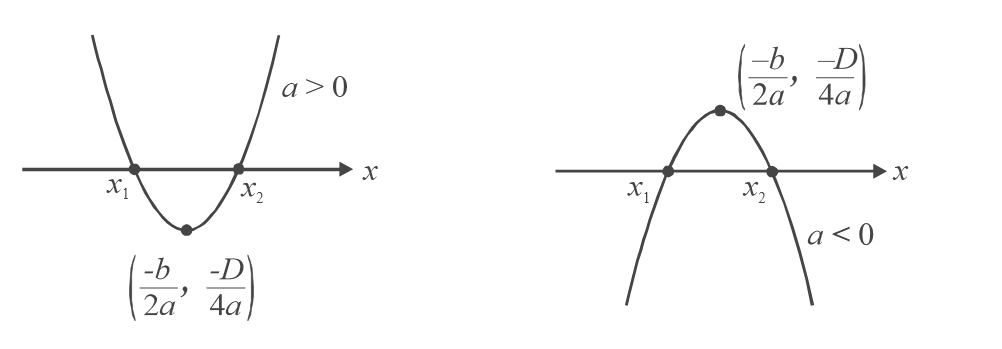Also, whether a > 0 or a < 0, the co-ordinates of the vertex are  \begin{align}\left( { - \frac{b}{{2a}},\frac{{ - D}}{{4a}}} \right)\end{align}  . What if a > 0 and D < 0?  The y-co-ordinate of the vertex, \begin{align}\frac{{ - D}}{{4a}}\end{align}  is positive, and hence the vertex lies above the  x-axis.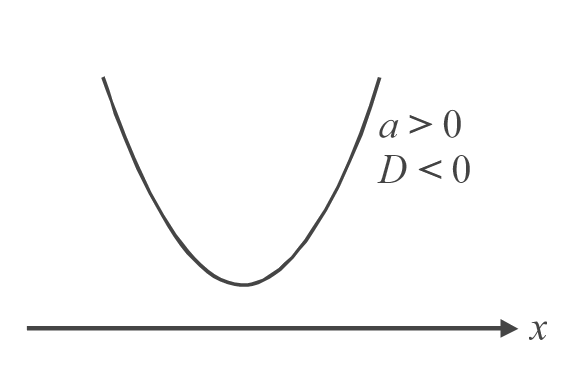f (x) in this case never cuts the axis and hence never becomes 0. We say that f(x) has no real roots. f(x) > 0 for all values of x.

Similarly, if a < 0 and D < 0, the y-co-ordinate of the vertex is negative and the graph lies below the axis.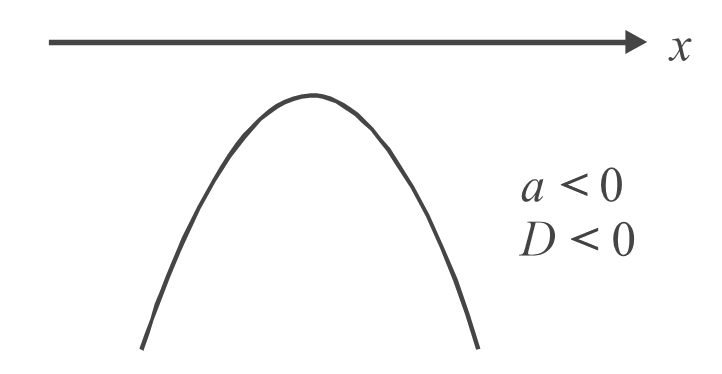Here also, f(x) has no real roots.

We see that whether a > 0 or a < 0, if D < 0, f(x) will not have real roots.

This is also evident in the formula for the roots in (ii). If D < 0, then $$\sqrt D$$  is imaginary (non-real).

What if a > 0 and D = 0? In this case, the y-coordinate of the vertex is 0 or the vertex lies on the  x-axis.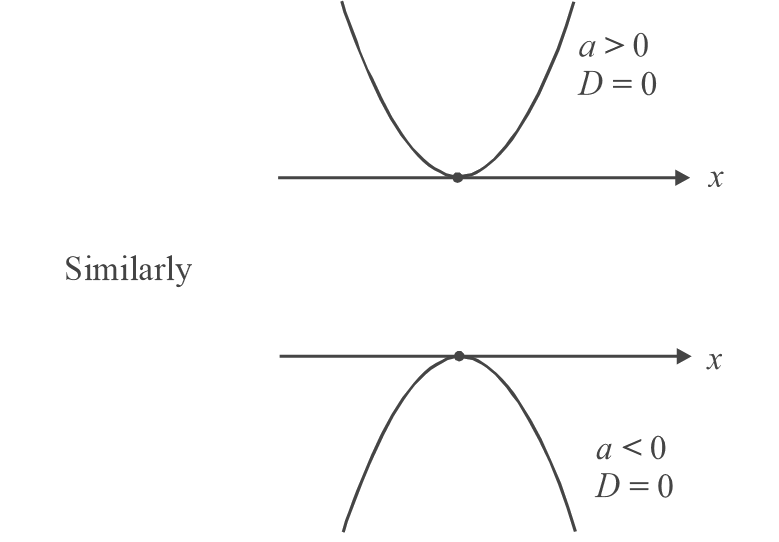Now we can easily determine the solutions to f(x) > 0 and f (x) < 0

All these results are summarized below.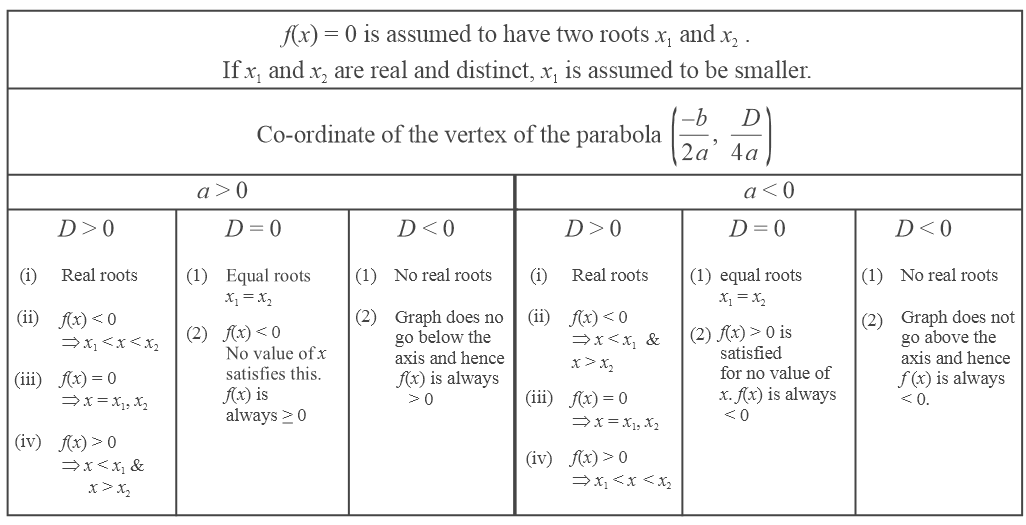You are urged not to memorize the results but understand them, by verifying each of them on your own

Learn from the best math teachers and top your exams

• Live one on one classroom and doubt clearing
• Practice worksheets in and after class for conceptual clarity
• Personalized curriculum to keep up with school